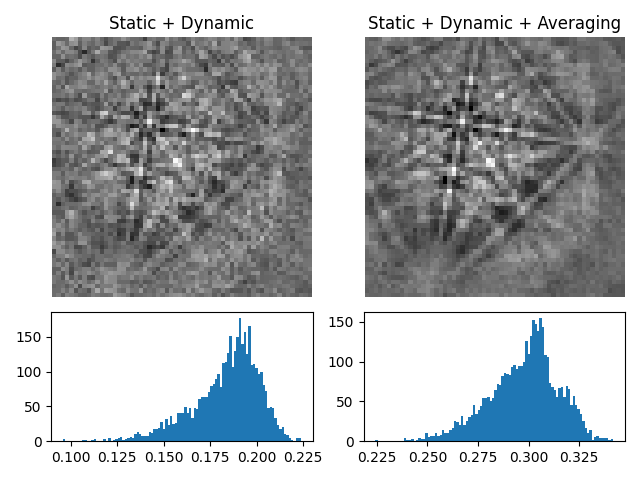# Neighbour pattern averaging#

This example shows how to average each pattern in a scan with its nearest neighbours using `average_neighbour_patterns()`.

More details are given in the pattern processing tutorial and the feature maps tutorial.```import hyperspy.api as hs
import kikuchipy as kp
import matplotlib.pyplot as plt

# Silence progressbars
hs.preferences.General.show_progressbar = False

# Load Ni patterns and subtract static and dynamic background
s = kp.data.nickel_ebsd_large()
s.remove_static_background()
s.remove_dynamic_background()

# Get image quality before averaging
iq0 = s.get_image_quality()

# Keep one pattern for comparison
x, y = (50, 8)
pattern0 = s.inav[x, y].deepcopy()

# Average in a (3, 3) window with a Gaussian kernel with a standard
# deviation of 1
s.average_neighbour_patterns(window="gaussian", std=1)

iq1 = s.get_image_quality()
pattern1 = s.inav[x, y]

# Plot pattern and histograms of image qualities before and after
# averaging
fig, axes = plt.subplots(2, 2, height_ratios=[3, 1.5])
for ax, pattern, title in zip(
axes,
[pattern0, pattern1],
["Static + Dynamic", "Static + Dynamic + Averaging"],
):
ax.imshow(pattern, cmap="gray")
ax.set_title(title)
ax.axis("off")
for ax, iq in zip(axes, [iq0, iq1]):
ax.hist(iq.ravel(), bins=100)
fig.tight_layout()
```

Total running time of the script: ( 0 minutes 5.792 seconds)

Estimated memory usage: 161 MB

Gallery generated by Sphinx-Gallery